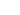# IF() Function

This article explains how to use IF() functions

The IF() function is arguably the most important function for building calculation forms. The basic use is if this, then that. The IF() function is perfect for enabling conditional logic in your form. The IF() function becomes even more powerful through a combination with other functions like AND() and OR() or chaining multiple IF() functions. Which is explained further in this doc.

Technically, the IF() function evaluates a condition and returns a value if it's TRUE and another value if it's FALSE.

``IF(logical_expression, value_if_true, value_if_false)``
• `logical_expression` - An expression or reference to a cell containing an expression that represents some logical value, i.e. TRUE or FALSE.
• `value_if_true` - The value the function returns if logical_expression is `TRUE`.
• `value_if_false` - [ OPTIONAL - blank by default ] - The value the function returns if logical_expression is `FALSE`.

In the formula builder your function will look like this:

``IF(QA > 50, QB * 2, "N/A")``
``IF(QA > QB, QA, QB)``

Notes:

• Ensure that value_if_true and value_if_false are provided to the function in the correct order - this is the single most common source of problems with IF
• Make sure there is no space between IF and the first bracket: `IF()` versus `IF ()`. The latter syntax will throw an error

# Example use

ConvertCalculator becomes really powerful when adding IF to a formula. Let's explain if by using an example:

I ask the user of two things:

• `QA`: The desired finish of the product (Silver or Gold)
• `QB`: Amount of products

Furthermore, we have the following requirements:

• The price of a silver product is \$ 100,-
• The price of a gold product is \$ 200,-
• If the total order value exceeds \$ 1,000,- we want to give a 5% discount

To calculate the total price we have the following formula:

`QA * QB`

To apply the discount we need to alter the formula using an IF statement:

``````IF(
QB * QB > 999,
QA * QB * 0.95,
QA * QB
)``````

# Chaining IF's

We can also chain IF-statements together. Let's build on top of the previous example. An important use case for chaining IF-statements is when you want to apply variable discounts. Let's add the following requirements:

• If the total order value exceeds \$ 1,500,- we want to give a 10% discount
• If the total order value exceeds \$ 2,500,- we want to give a 15% discount
• If the total order value exceeds \$ 5,000,- we want to give a 20% discount

We can easily accomplish this by chaining IF-statements in combination with the AND()-function. Also to keep our formula readable we'll make a variable for total price `VA` (`VA = QA * QB`). Our formula becomes:

``````IF(VA > 4999, VA * 0.80, 0) +
IF(AND(VA > 2499, VA < 5000), VA * 0.85, 0) +
IF(AND(VA > 1499, VA < 2500), VA * 0.90, 0) +
IF(AND(VA > 999, VA < 1500),  VA * 0.95, 0) +
IF(VA < 1000, VA, 0)``````

In plain language the formula says:

• if `total value` exceeds `4999` then `apply 20% discount on total price` otherwise `return zero`
• if `total value` is between `2499` AND `5000` then `apply 15% discount on total price` otherwise `return zero`
• if `total value` is between `1499` AND `2500` then `apply 10% discount on total price` otherwise `return zero`
• if `total value` is between `999` AND `1500` then `apply 5% discount on total price` otherwise `return zero`
• if `total value` is smaller than `1000` then provide `total price without a discount`

Notes:

• When returning numerical values use the `+` operator for chaining
• Your formula should end with a closing bracket. Accidentally putting a `+` at the end will throw an error
• When you have many conditionals that impact the formula, you are better off by using a datasheet and a more advanced formula like FINDIFS() or VLOOKUP().

If you want to also display the discount applied, you can create the following formula:

``````IF(VA > 4999, "20% discount", "") &
IF(AND(VA > 2499, VA < 5000), "15% discount", "") &
IF(AND(VA > 1499, VA < 2500), "10% discount", "") &
IF(AND(VA > 999, VA < 1500),  "5% discount", "") &
IF(VA < 1000, "No discount", "")``````

Note: since this formula is returning `string` values instead of `numerical` values, we use the `&` instead of the `+` and the `""` instead of the `0`.

# Using AND or OR

You can use AND or OR functions in a formula to make it even more powerful. Let's take the first example above, but add an extra finish to QA (platinum). The discount we want to take (when you buy more than 5 products) is not valuable on platinum. We need to use both AND and OR to accomplish this:

``````IF(
AND(QB > 5, OR(QA = 100, QA = 200)),
VA * 0.8,
VA
)``````

The above formula does this: If the product amount is greater than 5 and product finish is either silver or gold, apply the discount, else don't apply the discount.